### Home > MC2 > Chapter 11 > Lesson 11.1.1 > Problem11-6

11-6.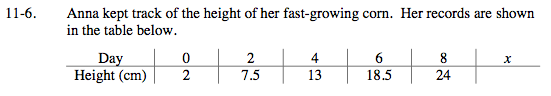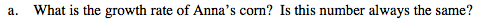Find the difference in height of Anna's corn from day 0 to day 2 to find
how much her corn has grown in 2 days.

7.5 − 2 = 5.5

Anna's corn grows 5.5 cm every 2 days.

To find how much Anna's corn grows in 1 day, divide that number by 2.

$\frac{5.5}{2} = 2.75$

The growth rate of Anna's corn is 2.75 cm per day.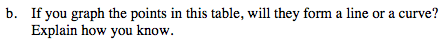If the growth rate of Anna's corn is constant, as concluded from (a),
what would a graph with constant growth look like?

If you need more help, graph the points on graphing paper.
Let the number of days be on the x-axis and the height in
centimeters be on the y-axis.

Since the rate of change is constant, the graph will form a line.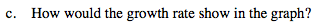What would the graph look like if the number of days was on the
x-axis, while the height in centimeters was on the y-axis?

If the y-coordinate increases by 2.75 for every day, the growth
rate will be represented by the slope of the line.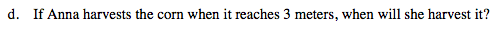3 meters is equivalent to how many centimeters?

3 meters is 300 centimeters. When will the
height (the y-coordinate) reach 300?

Find and use the rule to solve.